﻿ The Nexus between Market Tightness and Economic Growth – A Case of KenyaPublications are Open
Access in this journal
Article Versions
Export Article
• Normal Style
• MLA Style
• APA Style
• Chicago Style
Research Article
Open Access Peer-reviewed

### The Nexus between Market Tightness and Economic Growth – A Case of Kenya

Richard Wamalwa Wanzala , Willy Muturi, Tobias Olweny
Journal of Finance and Economics. 2017, 5(6), 259-268. DOI: 10.12691/jfe-5-6-2
Published online: October 25, 2017

### Abstract

This study investigates the nexus between the market tightness and economic growth in Kenya using data from 2006 – 2015 from Nairobi Security Exchange and Kenya National Bureau of Statistics. A parsimonious moderating regression analysis (MRA) has been presented to examine whether real interest rate and risk premium individually moderates the relationship between stock market tightness (proxied by various versions of spread) and the economic growth (proxied by Real Gross Domestic Product (GDP)) in Kenya. The study shows that both real interest rates and risk premium moderates the relationship between stock market tightness and the economic growth in Kenya.

### 1. Introduction

There has been momentous interest in both theoretical and empirical modeling of the behavior of market tightness because it’s critical dimension of liquidity that used in evaluating market's operational efficiency 8. For instance Chordia, et al. 9, examined a comprehensive sample of NYSE stocks that traded every day from 1993 through 2002 and pinpoints out that high liquidity facilitates arbitrage and leading to conclusion that liquidity plays an important role in efficiency creation. Further, market tightness elemental importance is demonstrated by the influence of trading costs on required returns which implies a direct linkage between liquidity and corporate costs of capital 10. A sound comprehension of key market tightness measures and their interaction with other macroeconomic variables increases investor confidence in the stock markets thereby catapulting efficacy of both individual investor and corporate resource allocation.

The growing body of research on market tightness primarily zero in on the stock markets in developed countries which are highly liquid 36. Conversely, this study shifts the attention from developed markets to an emerging market (that are characterized by poor liquidity) and specifically Kenya. Further, the initial empirical investigations were focused on the contemporaneous relationship between the broad-spectrum stock market liquidity measures and economic growth, and documented diverse results between these variables. For instance, Chogii, et al., 11, Chipaumire and Ngirande 12, Shatha 39, Olweny and Kimani 13, Nazir, et al. 1, Mohtadi and Argawal 38, and Levine and Zervos 14 found a positive relationship between stock market liquidity measures and the economic growth. For example, Levine and Zervos 14 empirical analysis of forty-two countries sampled over the period 1976-1993, illustrate that market liquidity is indeed positively and significantly correlated with measures of economic growth. On the other hand, Adigwe, et al. 15, Ifeoluwa and Motilewa 16, Wang and Ajit 17, Ngugi, et al. 18 and Alajekwu, et al. 35 researches indicate a negative relationship between stock market liquidity measures and the economic growth. Further, Wang and Ajit 17 empirical investigation on the impact of stock market development on economic growth in China using quarterly data from 1996 to 2011 and find that there is a negative relationship between the stock market liquidity and economic growth.

As a result, this study shifts from empirical analysis of conventional microstructure-based liquidity measures to in-depth analysis of market tightness proxied by three versions of spread; that is, log spread, log relative spread of log prices and adjusted log quote slope. More specifically, this study was directed towards determining the nexus between the market tightness and economic growth. The two null hypotheses that real interest rate does not moderate the market tightness and economic growth and risk premium does not moderate the market tightness and economic growth were also considered. Further, there is also momentous gain from utilizing annual data for market tightness in lieu of daily or monthly data; this is because neither daily nor monthly Real Gross Domestic Product (RGDP) data was not available.

### 2. Determination of Different Proxies of Market Tightness

The absolute spread (or quoted spread) can be regarded as the differences between the lowest ask price and the highest bid price. The absolute spread (absspread) was computed as: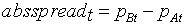(1)

where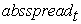is the absolute spread at time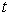,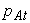is the lowest ask price and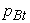is the highest bid price. Many authors, including with Chordia, et al. 10, Ranaldo 19, Grammig, et al., 20 Amihud, et al., 21 and Chiyachantana, et al. 22 showcase the use of absolute (quoted) spread and as a proxy for market liquidity. For instance, Chordia, et. al., 23 used absolute spread and effective spread measure in their study of liquidity and trading activity for a comprehensive sample of NYSE-listed stocks over an 11-year period. Similarly Grammig, et al., 20 use data from the German stock market and estimates the probability of informed trading for stocks that are simultaneously traded in a non-anonymous floor trading system and in an anonymous electronic auction market.

The log spread is absolute spread that has logarithmized to improve its distributional properties. In other words, it can be seen as simply as the differences of the logarithms of the lowest ask price and the highest bid price.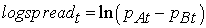(2)

where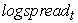is the log spread at time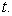The logspread is arithmetically easier to be used since its distribution properties are closer to a normal than the absolute spread. The logspread has been used by Hamao and Hasbrouck 24 and Zhang, et al., 8. For example, Zhang, et al., 8 uses logspread as one of the liquidity proxy to evaluate determinants of bid and ask quotes and implications for the cost of trading for intraday transactions. They use intraday data for the three major stocks for three month period of January 3, 2006 to March 31, 2006 for securities listed on NYSE.

The relative spread with the last trade (relspread) takes into consideration market dynamism whereby upward moving market have ask price as (pAt) whereas downward moving market have bid price (pBt). It’s important to note that pt must be established first before the ask price (pAt) and bid price (pBt) are quoted. Further, both relspread and transaction price would be irrelevant for actual market situation if the last transaction have a taken considerably long time before absspread is measured. Aldricha and Lee 25 and Loderer and Roth 26 use relative spread in their studies. The relspread is computed as: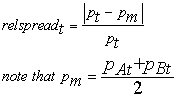(3)

where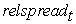is the relative spread at time t,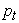is the last price of a security at time t. Aldricha and Lee 25 and Levin and Wright 27 used in relative spread in their researches.

2.4. Relative Spread of Log Prices

Relative spread of log prices (logrelspread) is computed akin to the logarithmic return of security. Like any other relative measures, it facilitates comparison between securities. The logrelspread is used to estimate liquidity by Hasbrouck and Seppi 28 after eliminating the time-of-day effects. The logrelspread is calculated as: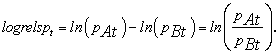(4)
2.4. Log Relative Spread of Log Prices

The absspread, logspread, relspread and logrelspread are characterized by strongly skewed distribution which complicates their computations. However, log relative spread of log prices (loglogrels) is much easier to be estimated through a normal distribution because it’s much more symmetrically distributed. Dacorogna, et al. 29 illustrates an introduction to the use of loglogrels in high frequency finance data. The loglogrels is estimated as: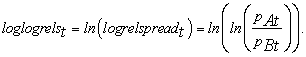(5)

The effective spread (effspread) is a measure of transaction costs associated with each trade, established by the difference between the execution price at market and the mid-quote on the market at a given time. Since execution of security is a function of transaction cost, the effective spreads are frequently constricted than time-weighted absspread. To calculate effective trade, the following formular is used: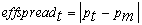(6)

where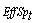is effective spread,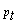is last trade of the month in the year t and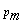is mid price. Chiyachantana, et al. 22 used effective spread and relative effective spread to examine the impact of regulation fair disclosure on liquidity, information asymmetry, and institutional and retail investors trading behavior. The researchers’ analyzed sample consists of all quarterly earnings announcements for NYSE stocks during an 18-month period from November 1, 1999 to July 31, 2001.

The relative spread (relspmidp) is sometimes known as inside spread and can be computed regardless whether last trade takes place or not. Most market liquidity researches have used relspmidp because it facilitates comparison of spread between different variables and it’s easier to compute. The relspmidp is computed as: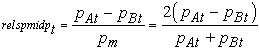where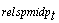is the relative spread,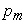is the mid price. Aldricha and Lee 25 and Levin and Wright 27 used in relative spread in their researches.

2.7. Log Quote Slope

Tightness can be determined using log quote slope (logqs) which is simply a logarithmized relative spread in the numerator of quote slope 28, 30. A decrease in the logqs means that the slope of the best quotes is better and the market is more liquid 30. Given that in the market the ask price () is always higher than the bid price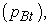the logqs are always positive. However as the difference betweenandtends to become narrower, the market also becomes more liquid. The same observation of the market becoming more liquid is made manifest with larger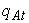and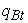respectively. The logqs is computed as follows: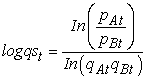(8)

where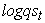is the log quote at a time t;was the best ask volume andwas the best bid volume at a time t;was the ask price andwas the bid price at a time t.

The adjusted log quote slope (logqsadj) was used but takes into account for the logqs for a market moving in one direction 31. Since then, logqsadj has been used as a measure of liquidity by various researchers 30, 32. The log quote slope is estimated as: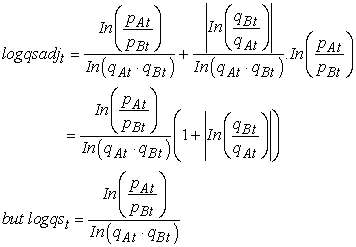(9a)

However, the log quote slope for time t-1 until time t is given by: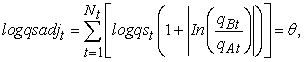(9b)

Where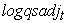is market tightness given by adjusted log quote slope at a time t;is the log quote at a time t;was the best ask volume andwas the best bid volume at a time t;was the ask price andwas the bid price at a time t.

### 3. Data and Methodology

A sagacious empirical execution to determine the how market liquidity impacts on economic growth is tricky to formulate because the ‘exact’ degree of liquidity is unobservable. However to examine the relationship between market tightness and economic growth, a Moderating Regression Analysis is applied. By using one predictor variable (interest rate and then risk premium), three separate regression equations are analyzed for egalitarianism of regression coefficients. As a result, an initial simple regression model is adopted as: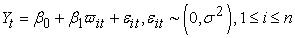(10)

where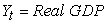at time t,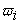is the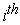proxy for market tightness at time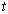from a sample size n,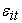is the random error term and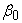and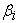are regression coefficients to be estimated. For robust inference, it is assumed that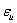has a mean of 0 and a variance of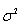and is uncorrelated with. However, when moderating variable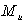enters the model, the following multiple regression equation (11) with additive predictor effect is borne: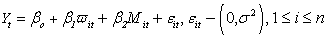(11)

whereis the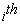moderating variable and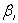on the other hand is the coefficient relating market tightness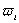to economic growth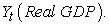Similarly,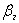is the coefficient relating the moderator variableto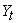. Finally, a multiple regression including interaction of predictors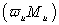was taken as: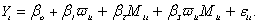(12)

The moderation effects is estimated by the product term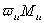and their associated coefficient of interaction; that is,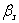. Ifis statistically different from zero, then it is concluded thatmoderates the relationship betweenand. Better still, the squared multiple correlation given by the interaction can be determined whether it is significantly greater than zero. Further, all(market tightness or predictor variables) and their interaction termare centered prior to model estimation not only to improve interpretation of regression coefficients but also to reduce the foreseeable multicollinearity incurred with interaction terms.

### 4. Empirical Results

4.1. Descriptive Statistics

The descriptive statistics for nine measures of market tightness and RGDP are illustrated in Table 1; that is, mean, median, maximum, minimum, standard deviation, skewness and kurtosis.

The mean value for RGDP is 5.25% with a positive skewness. The absspread has the highest mean of 2.185% than other measures of market tightness. On the other hand, relspmidp had the lowest mean of 0.004. Similarly, a critical analysis on standard deviation reveals that absspread and relspmidp have the highest and lowest variability as compared to other measures of market tightness demonstrating that the mean of these two variables must have pulled upwards and downwards respectively by extreme values. This statement is empirically supported from Table 1 whereby the maximum value of absspread of 15.17 is 56 times relative to low value of minima 0.27. Similarly, the maximum value of relspmidp of 0.026763 is 183 times the minima value of 0.0001459. All the liquidity measures have kurtosis less than three indicating that the distributions are not fat tailed in respect to normal distributions.

4.2. Normality Test

The Jarque–Bera tests (1980, 1987) was used to determine wether study varibles were normally distributed. The results of the normally tests were summarized in the following Table 2.

The the null hypothesis that sample data is not significantly different than a normal population was determined using Jarque–Bera test and the results summarized in the Table 2 above. From the Table 2, the null hypothesis of normality is accepted for Jarque–Bera test for all the market tightness variables under study because their calculated test statistic exceeded a critical value from the Chi-square distribution. That is, the test static values were between 0.2001 (for loglogrels) and 0.0001 (for absspread) were less than Chi-square distribution critical value of 5.99 with two degrees of freedom. This means that the data is normally distributed for all the study variables.

4.3. Correlation Matrix for Study Variables

Correlation is a measure that indicates direction and quantifies the strength of linear relationship between two quantitative variables 33. The correlation among different measures of liquidity has been analyzed in the past studies but a comprehensive overview in literature is lacking to date 31. This research used correlation analysis to determine the relationship among different market tightness proxies so as to reduce number as presented in Table 3.

4.4. Test for Stationarity

The ADF test was used to determine the stationarity between the dependent variable and the explanatory (market tightness) variables. Specifically, the ADF tests were estimated between RGDP and logqsadj; RGDP and loglogrels; RGDP and logspread; In essence, the null hypothesis that the series had a unit root was examined against the alternative hypothesis that series was stationary. Table 4 gives a summary of the results between RGDP and proxies of market tightness.

The tests for unit root level in 1, 5, and 10 percent critical τ values for RGDP were −4.803492, −3.403313 and −2.841819. Since the t value of −8.4730501 is more negative than any of these Mackinnon critical values, the conclusion is that the RGDP time series is stationary. This is consistent with p-value of 0.0011at 5%. Similary, the same conclusion was reached when testing for stationarity at first and second difference. For instance at the first difference, the ADF t statistic for RGDP was −8.473050 which was negative than any of these Mackinnon critical values of −4.803492, −3.403313 and −2.841819 at 1%, 5% and 10% respectively. On the other hand, the same results is also reflected for logqsadj, loglogrels and logspread; that is, these data are stationary at Level with p-values of 0.0010, 0.0042 and 0.0010 respectively.

4.5. Granger Causality Test

The most popular way to determine the causal relationships between two variables is through Granger causality test that was pioneered by Granger 7. Ever since its inception, the Granger causality test has been used widely in researches involving stock markets. The test is simple to carry out and involves use of bivariate vector autoregressions (VAR) between dependent and explanatory variable. In this study, the dependent variable was economic growth and explanatory vaiable was market tightness. The results of Granger causality are presented in Table 5.

The results in Table 5 suggests that there was no causality between LOGQSADJ and RGDP and vice versa since the estimated F for both tests was not significant at the 5% level whereby the Critical F value was 0.48010 and 0.70843 (for 1 lag and 9 degrees of freedom) respectively. This result leads to acceptance of null hypothesis that logqsadj does not Granger cause RGDP and RGDP does not Granger cause logqsadj. Nevertheless, research has indicated that the significance and direction of causality may depend critically on the number of lagged terms included. However, regardless the numbers of that are included; still the estimated F for both tests was not significant at the 5% level. Similarly, the results in Table 5 indicate non existence of causal relationship between RGDP and loglogrels and vice versa; and logspread and RGDP and vice versa. This is because the p-value for all tests was not significant at the 5% level; that is, p-value for all granger causality analysis is greater than 0.05.

4.6. Cointegration Test

Cointegration is defined as a formulation of the phenomenon that nonstationary processes can have linear combinations that are stationary 34. Johansen 34 further states that the components of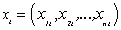are said to be cointegrated of order d, b denoted by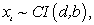if all components of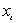are integrated of order d and there exists a vector of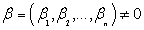such that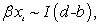where b> 0. For this study, the Unit Root tests for all the study variables affirmed that they were each stationary. As a result, a cointegration test was performed to determine whether the time series of these variables display a stationary process in a linear combination. Table 6 provides the Johansen-Juselius Cointegration test results. The lags interval (in first differences) was set 1 to 1 for all the study variables using the using the Schwarz Information Criterion SIC) so as to render the error term serially uncorrelated in conducting the test. Similarly, trace and maximal Eigenvalue statistics were adjusted by a factor (T-np)/T in order to correct bias towards finding evidence for cointegration in datasets. The effective number of observations in the study variables were given by T, n was the number of variables, and p was the lag order.

The results in Table 6 indicate that p-values of all the cointegration tests (that is Trace and Maximum Eigenvalue cointegration tests) were significant at 0.05 level thus leading to rejection of null hypothesis of no co-integration for these estimated models. For instance, the p-value for Unrestricted Cointegration Rank Test (Trace) for logqsadj and RGDP; loglogrels and RGDP; logspread and RGDP were all 0.0000 respectively. Similarly, the p-value for Unrestricted Cointegration Rank Test (Maximum Eigenvalue) was 0.0000, 0.0000 and 0.0000 respectively.

4.7. Moderated Regression Analysis (MRA)
4.7.1. MRA between Market Liquidity and Real Interest Rates (RealIR)

MRA was tested to investigate the null hypothesis that real interest rates do not moderate the relationship between stock market tightness and the economic growth in Kenya. After centering real interest rates, stock market tightness and the economic growth and computing interaction term, the two predictors and the interaction were entered into a simultaneous regression model. The results obtained are summarized in Table 7.

The R column in Table 7 indicates the association between the main effects of the predictors (market liquidity and real interest rate (RealIR)) and the RGDP for all the three models (A, B and C) for 1 and 2. The R2 for Model À1 is 84.1% and for the Model A2 is 99.7%. Thus, 84.1% in the variation of the RGDP is explained by the Model A1 and 99.7% in the variation of the RGDP is explained by the Model A2. Similarly, Adjusted R2, a more conservative estimate of model fit, is 79.6% and 99.6% for Model A1 and Model A2 respectively. Further, the R2 for Model B1 and Model C1 is 88.6% and 76.9% and for the Model B2 and Model C2 is 99.7% and 79.1% respectively. Thus, 88.6% in the variation of the RGDP is explained by the Model B1 and 99.7% in the variation of the RGDP is explained by the Model B2. Similarly, 76.9% in the variation of the RGDP is explained by the Model C1 and 79.1% in the variation of the RGDP is explained by the Model C2. Model A2, Model B2 and Model C2 standard error is 0.0095, 0.0097 and 0.2692 respectively. These standard errors for second regression models is lower than for first regression models which are 0.0663, 0.0582 and 1.1568 for Model A1, Model B1 and Model C1 respectively. Since the larger the standard error of the estimate the more error in a regression model, then the results depicts for second regression models as being better than first regression models.

The change statistics for first regression models is not significant for interpretation of the results because it is comparing Model A1, Model B1 and Model C1 with an empty model (i.e., no predictors) resulting in a value equal to the R2 for all the three models. However for second regression models, there is addition of the interaction term useful in determining the improvement in the model fit. From Table 7, Model A2 with the interaction between logqsadj and interest rates (Z1 =logqsadj*RealIR) accounted for significantly more variance than just logqsadj and RealIR by themselves (R2 change = 0.024, p-value = 0.000). Similarly, Model B2 with the interaction between loglogrels and interest rates (Z2 =loglogrels*RealIR) accounted for significantly more variance than just loglogrels and RealIR by themselves (R2 change = 0.112, p-value = 0.000). Finally, Model C2 with the interaction between logabsprd and interest rates (Z3 =logabsprd*RealIR) accounted for significantly more variance than just loglogrels and interest rates by themselves (R2 change = 0.313, p-value = 0.024). Since logqsadj, loglogrels and logabsprd are all proxies for market tightness; the results show that there is potentially significant moderation between market tightness and economic growth by real interest rates. In other words, we accept the alternative hypothesis that real interest rates moderate the relationship between stock market tightness and the economic growth in Kenya.

4.7.2. MRA between Market Liquidity and Risk Premium (RSKPRM)

MRA was tested to investigate the null hypothesis that risk premium (RSKPRM) moderate the relationship between stock market tightness and the economic growth in Kenya. After centering risk premium, stock market tightness and the economic growth and computing interaction term, the two predictors and the interaction were entered into a simultaneous regression model. The results obtained are summarized in Table 7.

The results for first regression models for Model D1, Model E1 and Model F1 in Table 8 are similar to the results for Model A1, Model B1 and Model C1 in Table 7 respectively. However, the results for second regression equations are different because the moderating variables are different; that is, RealIR for Table 7 and RSKPRM for Table 8 respectively. For instance, the R for second regression Model D2, Model E2 and Model F2 is 99.9%, 99.8% and 91.4% respectively. On the other hand, the R2 for the second regression Model D2, Model E2 and Model F2 is 99.9%, 99.7% and 83.5%. Thus, 99.9%, 99.7% and 83.5% in the variation of the RGDP is explained by the Model D2, Model E2 and Model F2 respectively. Further, adjusted R2 is 99.8%, 99.5% and 75.2% for Model E2, Model F2 and Model G2 respectively. The second regression Model D2, Model E2 and Model F2 standard error is 0.0061, 0.0099 and 0.2395 respectively. These values are lower than standard error 0.0663, 0.05821 and 1.1568 for corresponding first regression Model D1, Model E1 and Model F1. In essence, since the larger the standard error of the estimate the more error in a regression model, then the results depicts second regression models as being better than first regression models.

The change statistics for first regression Model D1, Model E1 and Model F1 is irrelevant for interpretation of the results because this entire three models it has no predictors. Thus, the R2 change for all the three models for instance, has a value equal to the R2. However for second regression Model D2, Model E2 and Model F2, there is addition of the interaction term (that is, Z4, Z5 and Z6) useful in determining the improvement in the model fit. Thus, Model D2 with the interaction between logqsadj and RISKPRM (Z4) accounted for significantly more variance than just logqsadj and RISKPRM by themselves i.e. R2 change = 0.158, p-value = 0.000. This result is consistent with Model E2 and Model F2 change of 0.459 and 0.389 and p-value of 0.000 and 0.002 respectively. These results fail to reject the hypothesis that risk premium moderates the relationship between stock market tightness and the economic growth in Kenya. Essentially, we accept the alternative hypothesis that real interest rates moderate the relationship between stock market tightness and the economic growth in Kenya.

### 5. Discussions

Most of the research on the relationship between stock market liquidity and economic growth does not use market tightness proxies. Majority of these studies use market depth proxies like capitalization and turn over ratios (for example, Nazir, et al. 1; Chipaumire and Ngirande 12; Shatha 39; Levin and Zervos 14 among others). Nevertheless, the results for Model A1, Model B1 and Model C1 (in Table 7) and Model D1, Model E1 and Model F1 (in Table 8) are consistent with similar studies but with different proxies of market liquidity (Nazir, et al. 1; Chipaumire and Ngirande 12 among others). In all this studies, it is established that market liquidity is indeed positively related with economic growth. However, their researches use measures of capitalization and turn over ratios as measures of stock market liquidity. On the other hand, it was difficult to relate the results of Model A2, Model B2 and Model C2 (in Table 7) and Model D2, Model E2 and Model F2 (in Table 8) with other research findings because past researches on market tightness focuses on different aspects. For instance, Chiyachantana, et al., 2004 and Ranaldo, 2006 focuses on the relationship between spreads (market tightness) around information symmetry and public information arrivals respectively. Table 7 presents results testing the null hypothesis that real interest rates does not significantly moderate the relationship between market tightness and economic growth where Table 8 presents results testing the null hypothesis that risk premium does not significantly moderate the relationship between market tightness and economic growth.

### 6. Conclusions

This study examined whether stock market tightness moderates the relationship between stock market tightness and the economic growth in Kenya. Using annual data from 2006 – 2015 from Nairobi Security Exchange and Kenya National Bureau of Statistics, MRA reveals that is potentially significant moderation between market tightness and economic growth by real interest rates. In other words, real interest rate moderates the relationship between stock market tightness and the economic growth in Kenya. Similarly, there is ample evidence that risk premium moderates the relationship between stock market tightness and the economic growth in Kenya.

The findings of this study are significant to policy makers to formulate policies that reduce spread so as to increase the propensity to trade on stocks on the trading floors, facilitate more efficient allocation of investment funds and fuel long-term economic growth. Needless to say, these policies will go a long way to avail more liquidity to different market participants at the stocks markets. One direction for future studies is to investigate the relationship between market tightness and economic growth by use of moderated mediation model (MMM) with a multiple mediators. The covariates may also be included in MMM to statistically account for shared associations between variables in the economic system of a given country caused by other macroeconomic variables. The covariates might be useful especially for cross countries studies to control for country-specific confounding or epiphenomenal associations between mediators and the economic growth.

### Acknowledgements

The research is funded by the authors.

### Statement of Competing Interests

The authors have no competing interests.

### References

Published with license by Science and Education Publishing, Copyright © 2017 Richard Wamalwa Wanzala, Willy Muturi and Tobias OlwenyThis work is licensed under a Creative Commons Attribution 4.0 International License. To view a copy of this license, visit http://creativecommons.org/licenses/by/4.0/